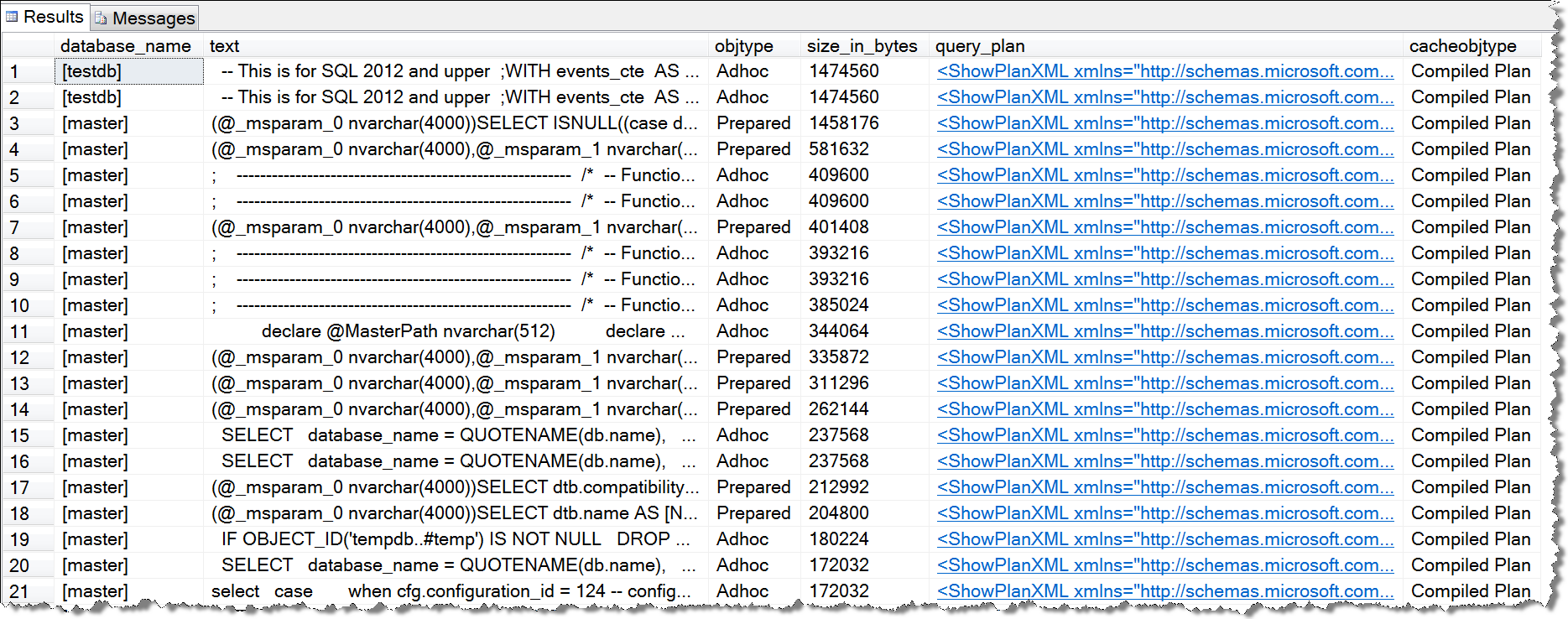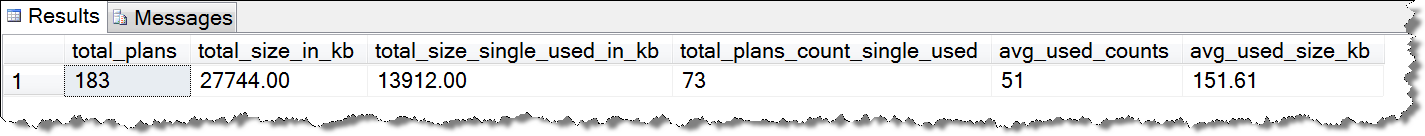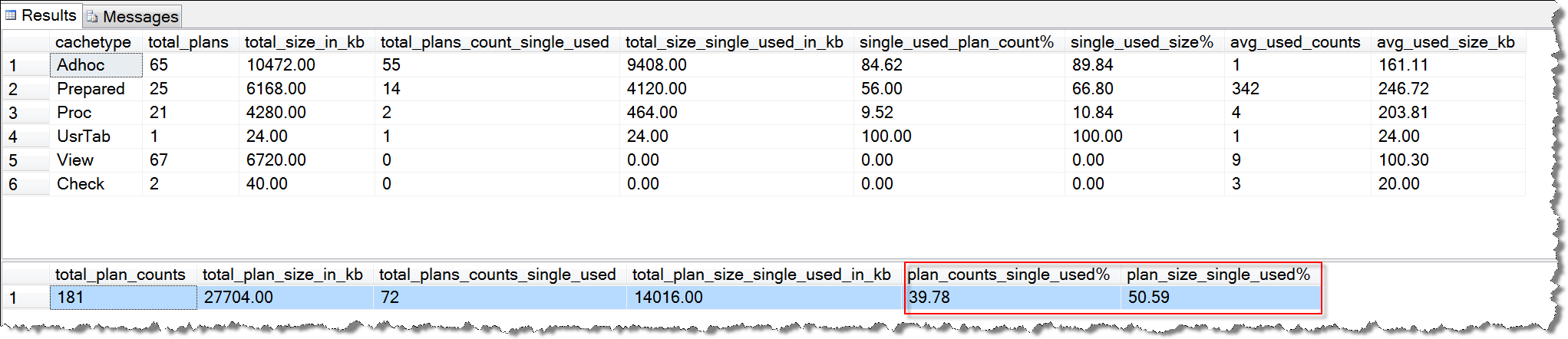2017/07/05 15:19

# 如何发现Single-used Plans

``````USE master
GO

SELECT
database_name = QUOTENAME(db.name),
st.text,
cp.objtype,
cp.size_in_bytes,
qp.query_plan,
cp.cacheobjtype
FROM sys.dm_exec_cached_plans AS cp
CROSS APPLY sys.dm_exec_sql_text(cp.plan_handle) st
INNER JOIN sys.databases AS db WITH(NOLOCK)
ON st.dbid = db.database_id
CROSS APPLY sys.dm_exec_query_plan(cp.plan_handle) AS qp
WHERE cp.cacheobjtype = N'Compiled Plan'
AND cp.usecounts = 1
ORDER BY cp.size_in_bytes DESC
``````# 执行计划缓存中有多少Single-used Plans

Single-used plans记录总数
Single-used plans总的执行计划占用的内存空间大小

``````USE master
GO
SELECT
COUNT(1) AS [total_plans],
CAST(SUM(size_in_bytes)/1024. AS DECIMAL(18,2)) AS [total_size_in_kb],
CAST(SUM((CASE WHEN usecounts = 1 THEN size_in_bytes ELSE 0 END))/1024. AS DECIMAL(12,2)) AS [total_size_single_used_in_kb],
SUM(CASE WHEN usecounts = 1 THEN 1 ELSE 0 END) AS [total_plans_count_single_used],
AVG(usecounts) AS [avg_used_counts],
CAST(AVG(size_in_bytes)/1024. AS DECIMAL(12, 2)) AS [avg_used_size_kb]
FROM sys.dm_exec_cached_plans WITH(NOLOCK)
``````# Single-used Plans对性能的影响

## 定性分析

总的执行计划缓存利用率不高：因为存在大量不能被重复利用的执行计划缓存，从而拉低了执行计划缓存整体利用率
浪费执行计划缓存中内存的开销：每一条执行计划缓存或多或少会占用一定的执行计划缓存内存空间，大量的Single-used Plans导致了内存空间的浪费
导致CPU使用率的上升：每一条SQL语句执行计划的编译、最优路径选择和执行计划缓存，这些过程都需要消耗系统CPU资源，如果大量存在Single-used Plans，会导致系统CPU使用率的上升。

执行计划缓存利用率就是0%，因为没有任何的执行计划被重用
执行计划缓存这种设计就毫无意义，因为缓存起来也没有被重用
浪费执行计划缓存的内存开销和CPU开销

## 定量分析

``````USE master
GO

IF OBJECT_ID('tempdb..#temp') IS NOT NULL
DROP TABLE #temp

SELECT
objtype AS [cachetype],
COUNT(1) AS [total_plans],
CAST(SUM(size_in_bytes)/1024. AS DECIMAL(18,2)) AS [total_size_in_kb],
CAST(SUM((CASE WHEN usecounts = 1 THEN size_in_bytes ELSE 0 END))/1024. AS DECIMAL(12,2)) AS [total_size_single_used_in_kb],
SUM(CASE WHEN usecounts = 1 THEN 1 ELSE 0 END) AS [total_plans_count_single_used],
AVG(usecounts) AS [avg_used_counts],
CAST(AVG(size_in_bytes)/1024. AS DECIMAL(12, 2)) AS [avg_used_size_kb]
INTO #temp
FROM sys.dm_exec_cached_plans WITH(NOLOCK)
GROUP BY objtype

SELECT
[cachetype],
[total_plans],
[total_size_in_kb],
[total_plans_count_single_used],
[total_size_single_used_in_kb],
CAST(([total_plans_count_single_used]*1.0 / [total_plans]*1.0) * 100. AS DECIMAL(12, 2)) AS [single_used_plan_count%],
CAST([total_size_single_used_in_kb]/[total_size_in_kb] * 100. AS DECIMAL(12, 2)) AS [single_used_size%],
[avg_used_counts],
[avg_used_size_kb]
FROM #temp
ORDER BY [total_size_single_used_in_kb] DESC

SELECT
SUM([total_plans]) AS total_plan_counts,
SUM([total_size_in_kb]) AS total_plan_size_in_kb,
SUM([total_plans_count_single_used]) AS [total_plans_counts_single_used],
SUM([total_size_single_used_in_kb]) AS [total_plan_size_single_used_in_kb],
CAST(SUM([total_plans_count_single_used]) * 100. / SUM([total_plans]) AS DECIMAL(12, 2)) AS [plan_counts_single_used%],
CAST(SUM([total_size_single_used_in_kb]) * 100. / SUM([total_size_in_kb]) AS DECIMAL(12, 2)) AS [plan_size_single_used%]
FROM #temp
``````# 解决Single-used Plans问题

## 清空Single-used Plans

``````USE master
GO

DECLARE
@plan_handle varbinary(64)
;

DECLARE
cur_single_used_plan_handle CURSOR LOCAL STATIC READ_ONLY FORWARD_ONLY
FOR
SELECT cp.plan_handle
FROM sys.dm_exec_cached_plans AS cp WITH(NOLOCK)
WHERE usecounts = 1

OPEN cur_single_used_plan_handle
FETCH NEXT FROM cur_single_used_plan_handle INTO @plan_handle
WHILE @@FETCH_STATUS = 0
BEGIN
PRINT @plan_handle

DBCC FREEPROCCACHE (@plan_handle);

FETCH NEXT FROM cur_single_used_plan_handle INTO @plan_handle
END

CLOSE cur_single_used_plan_handle
DEALLOCATE cur_single_used_plan_handle
GO
``````

##0
0 收藏

### 作者的其它热门文章0 评论
0 收藏
0